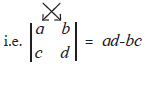# Determinant of Order Two

#### notes

|[a,b],[c,d]| is a determinant. (a, b), (c, d) are rows and ([a],[c]), ([b],[d]) are columns.

Degree of this determinant is 2, because there are 2 elements in each column and 2 elements in each row. Determinant represents a number which is (ad-bc).ad-bc is the value of determinant |[a,b],[c,d]|

Determinants, usually, are represented with capital letters as A, B, C, D, . . . . . etc.

Example: Find the values of the following determinant.

A=|[5,3],[7,9]|

Solution:

A=|[5,3],[7,9]| = (5xx9)-(3xx7)=45-21=24

If you would like to contribute notes or other learning material, please submit them using the button below.

#### Video Tutorials

We have provided more than 1 series of video tutorials for some topics to help you get a better understanding of the topic.

Series 1

Series 2

### Shaalaa.com

Simultaneous Equation : Determinant Method (Cramer's Rule). [00:08:47]
S
0%